Home Practice
For learners and parents For teachers and schools
Textbooks
Full catalogue
Pricing SupportLog in

We think you are located in United States. Is this correct?

# Chapter 3: Atomic combinations

In this chapter learners will explore the concept of a covalent bond in greater detail. In grade ten learners learnt about the three types of chemical bond (ionic, covalent and metallic). A great video to introduce this topic is: Veritasium chemical bonding song. In this chapter the focus is on the covalent bond. A short breakdown of the topics in this chapter follows.

• Electron structure and Lewis diagrams (from grade $$\text{10}$$)

As revision you can ask learners to draw Lewis diagrams for the first $$\text{20}$$ elements and give the electronic structure (this was covered in grade $$\text{10}$$). This then leads into thinking how the elements can share electrons in a bond. Learners should recognise that there are unpaired electrons in atoms that can be shared to form the bonds.

It is important to note that when drawing Lewis diagrams, we first place single electrons around the central atom and only once four electrons have been placed, do we pair electrons up. This will avoid the need to explain hybridisation. It is also important for learners to realise that the placement of electrons is arbitrary and the electrons can be placed anywhere around the atom.

• Why hydrogen is a diatomic molecule but helium is a monatomic molecule

This part of the chapter is interleaved with electron structure and Lewis diagrams as these two concepts play a key role in understanding why hydrogen is diatomic and helium is monatomic. In this part learners are introduced to the idea that when two atoms come close together there is a change in the potential energy. This forms a strong foundation for explaining the energy changes that occur in chemical reactions and will be seen again in chapter $$\text{12}$$ (energy changes in chemical reactions).

• Deducing simple rules about bond formation (and drawing Lewis diagrams for these molecules)

Four cases are looked at to try to understand why bonds form. This is all about the covalent bond, so all the examples you use must be of covalent molecules (and you must also only pick examples of covalent molecular structures as covalent network structures are more like ionic networks and do not form simple molecular units). It is also important to help learners realise that a lone pair of electrons is very much dependant on the molecule that they are looking at. Lone pairs of electrons can be used under special circumstances to form dative (or coordinate) covalent bonds.

• The basic principles of VSEPR and predicting molecular shape

You can build the different molecular shapes before starting to teach VSEPR from large polystyrene balls and kebab sticks or you can give your learners jellytots or marshmallows and toothpicks and get them to build the molecular shapes. Remember that the shapes with lone pairs need more space for the lone pairs and so it is not as simple as just removing the toothpick for the lone pair.

This topic covers the shapes that molecules have. This is only the shapes of covalent molecular compounds, covalent network structures, ionic compounds and metals have very different three dimensional forms. This topic is important to help learners determine polarity of molecules. Two approaches are used to determine the shape of a molecule. The first one looks at molecules matching up to a general formula while the second one considers how many electron pairs are around a central atom. These two approaches can be used together to help learners fully understand this topic.

• Electronegativity and polarity of bonds

It is important to note that CAPs does not give a definitive source for electronegativity values. You should use the ones found on the periodic table in the matric exams (these are the same values as the ones on the periodic table at the front of this book). Learners should be aware that they may see different values on other periodic tables. Learners must not think of the different types of bonding as being exactly defined. Also, the values for where the types of bonding transition are not exact and different sources quote different cut-off points.

The simplest examples of polarity are the ideal shapes with all the end atoms the same and so you should stick to this in your explanation. You can explain this for trigonal planar molecules by using your learners. Get three girls or three boys to link hands (they all put their right hand into the centre and hold the other two learners right hands). Then they try to pull away (all learners pull equally). This is the even sharing of electrons. Now replace a girl with a boy (or vice versa) and tell the new learner to pull a bit less. This shows the uneven sharing of electrons.

• Bond length and bond energy

In this final part of the chapter we return to our energy diagram and add two pieces of information: bond energy and bond length. The bond length is the distance between the two atoms when they are at their minimum energy, while the bond energy is this minimum energy. The bond energy comes up again in chapter $$\text{12}$$ (energy and chemical change) when the topic of exothermic and endothermic reactions is covered.

Coloured text has been used as a tool to highlight different parts of Lewis diagrams. Ensure that learners understand that the coloured text does not mean there is anything special about that part of the diagram, this is simply a teaching tool to help them identify the important aspects of the diagram, in particular the unpaired electrons.

We live in a world that is made up of many complex compounds. All around us we see evidence of chemical bonding from the chair you are sitting on, to the book you are holding, to the air you are breathing. Imagine if all the elements on the periodic table did not form bonds but rather remained on their own. Our world would be pretty boring with only $$\text{100}$$ or so elements to use.

Imagine you were painting a picture and wanted to show the colours around you. The only paints you have are red, green, yellow, blue, white and black. Yet you are able to make pink, purple, orange and many other colours by mixing these paints. In the same way, the elements can be thought of as natures paint box. The elements can be joined together in many different ways to make new compounds and so create the world around you.

In Grade $$\text{10}$$ we started exploring chemical bonding. In this chapter we will go on to explain more about chemical bonding and why chemical bonding occurs. We looked at the three types of bonding: covalent, ionic and metallic. In this chapter we will focus mainly on covalent bonding and on the molecules that form as a result of covalent bonding.

In this chapter we will use the term molecule to mean a covalent molecular structure. This is a covalent compound that interacts and exists as a single entity.

## 3.1 Chemical bonds (ESBM4)

### Why do atoms bond? (ESBM5)

As we begin this section, it's important to remember that what we will go on to discuss is a model of bonding, that is based on a particular model of the atom. You will remember from the discussion on atoms (in Grade $$\text{10}$$) that a model is a representation of what is happening in reality. In the model of the atom that you are learnt in Grade $$\text{10}$$, the atom is made up of a central nucleus, surrounded by electrons that are arranged in fixed energy levels (sometimes called shells). Within each energy level, electrons move in orbitals of different shapes. The electrons in the outermost energy level of an atom are called the valence electrons. This model of the atom is useful in trying to understand how different types of bonding take place between atoms.

A model takes what we see in the world around us and uses that to make certain predictions about what we cannot see.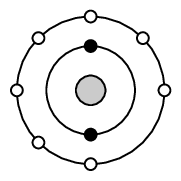Figure 3.1: Electron arrangement of a fluorine atom. The black electrons (small circles on the inner ring) are the core electrons and the white electrons (small circles on the outer ring) are the valence electrons.

The following points were made in these earlier discussions on electrons and energy levels:

• Electrons always try to occupy the lowest possible energy level.
• The noble gases have a full valence electron orbital. For example neon has the following electronic configuration: $$1\text{s}^{2}2\text{s}^{2}2\text{p}^{6}$$. The second energy level is the outermost (valence) shell and is full.
• Atoms form bonds to try to achieve the same electron configuration as the noble gases.
• Atoms with a full valence electron orbital are less reactive.

temp text

### Energy and bonding (ESBM6)

There are two cases that we need to consider when two atoms come close together. The first case is where the two atoms come close together and form a bond. The second case is where the two atoms come close together but do not form a bond. We will use hydrogen as an example of the first case and helium as an example of the second case.

#### Case 1: A bond forms

Let's start by imagining that there are two hydrogen atoms approaching one another. As they move closer together, there are three forces that act on the atoms at the same time. These forces are described below:

1. repulsive force between the electrons of the atoms, since like charges repel

2. attractive force between the nucleus of one atom and the electrons of another

3. repulsive force between the two positively-charged nuclei

These three forces work together when two atoms come close together. As the total force experienced by the atoms changes, the amount of energy in the system also changes.

Now look at Figure 3.5 to understand the energy changes that take place when the two atoms move towards each other.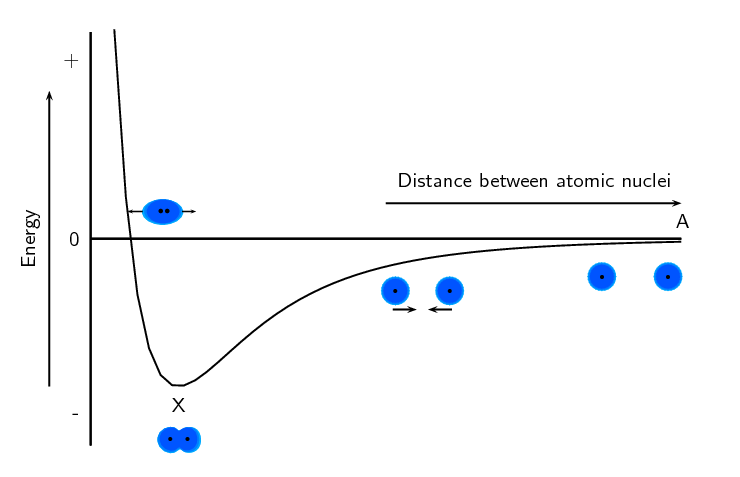Figure 3.5: Graph showing the change in energy that takes place as two hydrogen atoms move closer together.

Let us imagine that we have fixed the one atom and we will move the other atom closer to the first atom. As we move the second hydrogen atom closer to the first (from point A to point X) the energy of the system decreases. Attractive forces dominate this part of the interaction. As the second atom approaches the first one and gets closer to point X, more energy is needed to pull the atoms apart. This gives a negative potential energy.

At point X, the attractive and repulsive forces acting on the two hydrogen atoms are balanced. The energy of the system is at a minimum.

Further to the left of point X, the repulsive forces are stronger than the attractive forces and the energy of the system increases.

For hydrogen the energy at point X is low enough that the two atoms stay together and do not break apart again. This is why when we draw the Lewis diagram for a hydrogen molecule we draw two hydrogen atoms next to each other with an electron pair between them.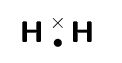We also note that this arrangement gives both hydrogen atoms a full outermost energy level (through the sharing of electrons or covalent bonding).

#### Case 2: A bond does not form

Now if we look at helium we see that each helium atom has a filled outer energy level. Looking at Figure 3.6 we find that the energy minimum for two helium atoms is very close to zero. This means that the two atoms can come together and move apart very easily and never actually stick together.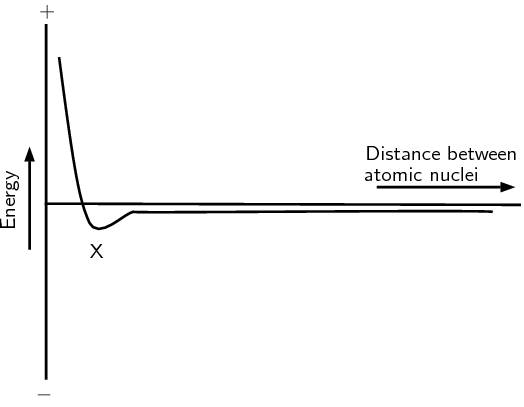Figure 3.6: Graph showing the change in energy that takes place as two helium atoms move closer together.

For helium the energy minimum at point X is not low enough that the two atoms stay together and so they move apart again. This is why when we draw the Lewis diagram for helium we draw one helium atom on its own. There is no bond.

We also see that helium already has a full outermost energy level and so no compound forms.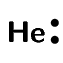### Valence electrons and Lewis diagrams (ESBM7)

Now that we understand a bit more about bonding we need to refresh the concept of Lewis diagrams that you learnt about in Grade $$\text{10}$$. With the knowledge of why atoms bond and the knowledge of how to draw Lewis diagrams we will have all the tools that we need to try to predict which atoms will bond and what shape the molecule will be.

In grade $$\text{10}$$ we learnt how to write the electronic structure for any element. For drawing Lewis diagrams the one that you should be familiar with is the spectroscopic notation. For example the electron configuration of chlorine in spectroscopic notation is: $$1\text{s}^{2}2\text{s}^{2}2\text{p}^{5}$$. Or if we use the condensed form: $$[\text{He}]2\text{s}^{2}2\text{p}^{5}$$. The condensed spectroscopic notation quickly shows you the valence electrons for the element.

Using the number of valence electrons we can easily draw Lewis diagrams for any element. In Grade $$\text{10}$$ you learnt how to draw Lewis diagrams. We will refresh the concepts here as they will aid us in our discussion of bonding.

A Lewis diagram uses dots or crosses to represent the valence electrons on different atoms. The chemical symbol of the element is used to represent the nucleus and the core electrons of the atom.

Lewis diagrams for the elements in period $$\text{2}$$ are shown below:

 Element Group number Valence electrons Spectroscopic notation Lewis diagram Lithium $$\text{1}$$ $$\text{1}$$ $$[\text{He}]2\text{s}^{1}$$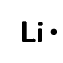Beryllium $$\text{2}$$ $$\text{2}$$ $$[\text{He}]2\text{s}^{2}$$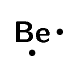Boron $$\text{13}$$ $$\text{3}$$ $$[\text{He}]2\text{s}^{2}2\text{p}^{1}$$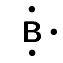Carbon $$\text{14}$$ $$\text{4}$$ $$[\text{He}]2\text{s}^{2}2\text{p}^{2}$$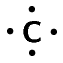Nitrogen $$\text{15}$$ $$\text{5}$$ $$[\text{He}]2\text{s}^{2}2\text{p}^{3}$$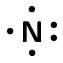Oxygen $$\text{16}$$ $$\text{6}$$ $$[\text{He}]2\text{s}^{2}2\text{p}^{4}$$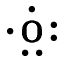Fluorine $$\text{17}$$ $$\text{7}$$ $$[\text{He}]2\text{s}^{2}2\text{p}^{5}$$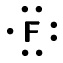Neon $$\text{18}$$ $$\text{8}$$ $$[\text{He}]2\text{s}^{2}2\text{p}^{6}$$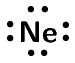You can place the unpaired electrons anywhere (top, bottom, left or right). The exact ordering in a Lewis diagram does not matter.

## Lewis diagrams

Textbook Exercise 3.1

Give the spectroscopic notation and draw the Lewis diagram for:

magnesium

$$[\text{Ne}]3\text{s}^{2}$$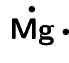sodium

$$[\text{Ne}]3\text{s}^{1}$$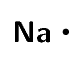chlorine

$$[\text{Ne}]3\text{s}^{2}3\text{p}^{5}$$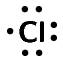aluminium

$$[\text{Ne}]3\text{s}^{2}3\text{p}^{1}$$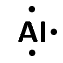argon

$$[\text{Ne}]3\text{s}^{2}3\text{p}^{6}$$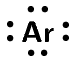### Covalent bonds and bond formation (ESBM8)

Covalent bonding involves the sharing of electrons to form a chemical bond. The outermost orbitals of the atoms overlap so that unpaired electrons in each of the bonding atoms can be shared. By overlapping orbitals, the outer energy shells of all the bonding atoms are filled. The shared electrons move in the orbitals around both atoms. As they move, there is an attraction between these negatively charged electrons and the positively charged nuclei. This attractive force holds the atoms together in a covalent bond.

Covalent bond
A form of chemical bond where pairs of electrons are shared between atoms.

Covalent bonds are examples of interatomic forces.

We will look at a few simple cases to deduce some rules about covalent bonds.

Remember that it is only the valence electrons that are involved in bonding, and so when diagrams are drawn to show what is happening during bonding, it is only these electrons that are shown. Dots or crosses represent electrons in different atoms.

#### Case 1: Two atoms that each have an unpaired electron

For this case we will look at hydrogen chloride and methane.

## Worked example 1: Lewis diagrams: Simple molecules

Represent hydrogen chloride ($$\text{HCl}$$) using a Lewis diagram.

### For each atom, determine the number of valence electrons in the atom, and represent these using dots and crosses.

The electron configuration of hydrogen is $$1\text{s}^{1}$$ and the electron configuration for chlorine is $$[\text{He}]2\text{s}^{2}2\text{p}^{5}$$. The hydrogen atom has $$\text{1}$$ valence electron and the chlorine atom has $$\text{7}$$ valence electrons.

The Lewis diagrams for hydrogen and chlorine are:Notice the single unpaired electron (highlighted in blue) on each atom. This does not mean this electron is different, we use highlighting here to help you see the unpaired electron.

### Arrange the electrons so that the outermost energy level of each atom is full.

Hydrogen chloride is represented below.Notice how the two unpaired electrons (one from each atom) form the covalent bond.

The dot and cross in between the two atoms, represent the pair of electrons that are shared in the covalent bond. We can also show this bond using a single line: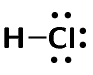Note how we still show the other electron pairs around chlorine.

From this we can conclude that any electron on its own will try to pair up with another electron. So in practise atoms that have at least one unpaired electron can form bonds with any other atom that also has an unpaired electron. This is not restricted to just two atoms.

## Worked example 2: Lewis diagrams: Simple molecules

Represent methane ($$\text{CH}_{4}$$) using a Lewis diagram

### For each atom, determine the number of valence electrons in the atom, and represent these using dots and crosses.

The electron configuration of hydrogen is $$1\text{s}^{1}$$ and the electron configuration for carbon is $$[\text{He}]2\text{s}^{2}2\text{p}^{2}$$. Each hydrogen atom has $$\text{1}$$ valence electron and the carbon atom has $$\text{4}$$ valence electrons.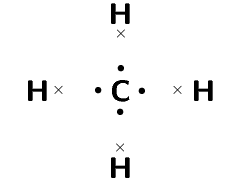Remember that we said we can place unpaired electrons at any position (top, bottom, left, right) around the elements symbol.

### Arrange the electrons so that the outermost energy level of each atom is full.

The methane molecule is represented below.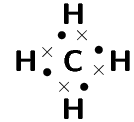Or: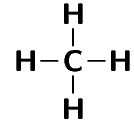Textbook Exercise 3.2

Represent the following molecules using Lewis diagram:

chlorine ($$\text{Cl}_{2}$$)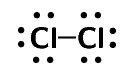boron trifluoride ($$\text{BF}_{3}$$)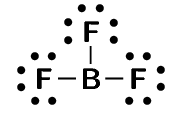temp text

#### Case 2: Atoms with lone pairs

We will use water as an example. Water is made up of one oxygen and two hydrogen atoms. Hydrogen has one unpaired electron. Oxygen has two unpaired electrons and two electron pairs. From what we learnt in the first examples we see that the unpaired electrons can pair up. But what happens to the two pairs? Can these form bonds?

## Worked example 3: Lewis diagrams: Simple molecules

Represent water ($$\text{H}_{2}\text{O}$$) using a Lewis diagram

### For each atom, determine the number of valence electrons in the atom, and represent these using dots and crosses.

The electron configuration of hydrogen is $$1\text{s}^{1}$$ and the electron configuration for oxygen is $$[\text{He}]2\text{s}^{2}2\text{p}^{4}$$. Each hydrogen atom has $$\text{1}$$ valence electron and the oxygen atom has $$\text{6}$$ valence electrons.### Arrange the electrons so that the outermost energy level of each atom is full.

The water molecule is represented below.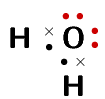or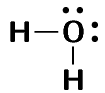Notice how in this example we wrote a $$\text{2}$$ in front of the hydrogen? Instead of writing the Lewis diagram for hydrogen twice, we simply write it once and use the $$\text{2}$$ in front of it to indicate that two hydrogens are needed for each oxygen.

And now we can answer the questions that we asked before the worked example. We see that oxygen forms two bonds, one with each hydrogen atom. Oxygen however keeps its electron pairs and does not share them. We can generalise this to any atom. If an atom has an electron pair it will normally not share that electron pair.

A lone pair is an unshared electron pair. A lone pair stays on the atom that it belongs to.

A lone pair can be used to form a dative covalent bond.

In the example above the lone pairs on oxygen are highlighted in red. When we draw the bonding pairs using lines it is much easier to see the lone pairs on oxygen.

Textbook Exercise 3.3

Represent the following molecules using Lewis diagrams:

ammonia ($$\text{NH}_{3}$$)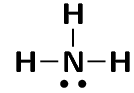oxygen difluoride ($$\text{OF}_{2}$$)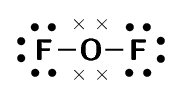temp text

#### Case 3: Atoms with multiple bonds

We will use oxygen and hydrogen cyanide as examples.

## Worked example 4: Lewis diagrams: Molecules with multiple bonds

Represent oxygen ($$\text{O}_{2}$$) using a Lewis diagram

### For each atom, determine the number of valence electrons that the atom has from its electron configuration.

The electron configuration of oxygen is $$[\text{He}]2\text{s}^{2}2\text{p}^{4}$$. Oxygen has $$\text{6}$$ valence electrons.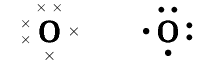### Arrange the electrons in the $$\text{O}_{2}$$ molecule so that the outermost energy level in each atom is full.

The $$\text{O}_{2}$$ molecule is represented below. Notice the two electron pairs between the two oxygen atoms (highlighted in blue). Because these two covalent bonds are between the same two atoms, this is a double bond.or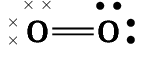Each oxygen atom uses its two unpaired electrons to form two bonds. This forms a double covalent bond (which is shown by a double line between the two oxygen atoms).

## Worked example 5: Lewis diagrams: Molecules with multiple bonds

Represent hydrogen cyanide ($$\text{HCN}$$) using a Lewis diagram

### For each atom, determine the number of valence electrons that the atom has from its electron configuration.

The electron configuration of hydrogen is $$1\text{s}^{1}$$, the electron configuration of nitrogen is $$[\text{He}]2\text{s}^{2}2\text{p}^{3}$$ and for carbon is $$[\text{He}]2\text{s}^{2}2\text{p}^{2}$$. Hydrogen has $$\text{1}$$ valence electron, carbon has $$\text{4}$$ valence electrons and nitrogen has $$\text{5}$$ valence electrons.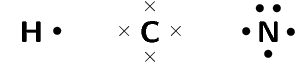### Arrange the electrons in the $$\text{HCN}$$ molecule so that the outermost energy level in each atom is full.

The $$\text{HCN}$$ molecule is represented below. Notice the three electron pairs (highlighted in red) between the nitrogen and carbon atom. Because these three covalent bonds are between the same two atoms, this is a triple bond.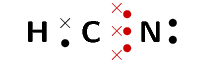or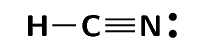As we have just seen carbon shares one electron with hydrogen and three with nitrogen. Nitrogen keeps its electron pair and shares its three unpaired electrons with carbon.

Textbook Exercise 3.4

Represent the following molecules using Lewis diagrams:

acetylene ($$\text{C}_{2}\text{H}_{2}$$)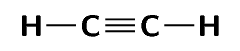formaldehyde ($$\text{CH}_{2}\text{O}$$)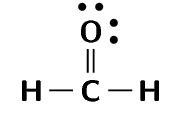temp text

#### Case 4: Co-ordinate or dative covalent bonds

Dative covalent bond
This type of bond is a description of covalent bonding that occurs between two atoms in which both electrons shared in the bond come from the same atom.

A dative covalent bond is also known as a coordinate covalent bond. Earlier we said that atoms with a pair of electrons will normally not share that pair to form a bond. But now we will see how an electron pair can be used by atoms to form a covalent bond.

One example of a molecule that contains a dative covalent bond is the ammonium ion ($$\text{NH}_{4}^{+}$$) shown in the figure below. The hydrogen ion $$\text{H}^{+}$$ does not contain any electrons, and therefore the electrons that are in the bond that forms between this ion and the nitrogen atom, come only from the nitrogen.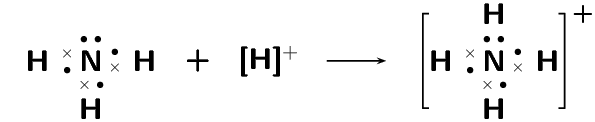Notice that the hydrogen ion is charged and that this charge is shown on the ammonium ion using square brackets and a plus sign outside the square brackets.

We can also show this as: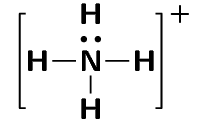Note that we do not use a line for the dative covalent bond.

Another example of this is the hydronium ion ($$\text{H}_{3}\text{O}^{+}$$).

To summarise what we have learnt:

• Any electron on its own will try to pair up with another electron. So in theory atoms that have at least one unpaired electron can form bonds with any other atom that also has an unpaired electron. This is not restricted to just two atoms.

• If an atom has an electron pair it will normally not share that pair to form a bond. This electron pair is known as a lone pair.

• If an atom has more than one unpaired electron it can form multiple bonds to another atom. In this way double and triple bonds are formed.

• A dative covalent bond can be formed between an atom with no electrons and an atom with a lone pair.

## Atomic bonding and Lewis diagrams

Textbook Exercise 3.5

Represent each of the following atoms using Lewis diagrams:

calcium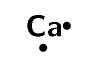lithium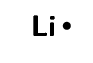phosphorous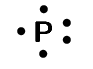potassium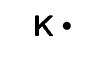silicon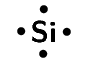sulfurRepresent each of the following molecules using Lewis diagrams:

bromine ($$\text{Br}_{2}$$)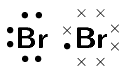carbon dioxide ($$\text{CO}_{2}$$)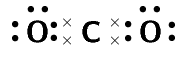nitrogen ($$\text{N}_{2}$$)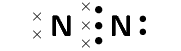hydronium ion ($$\text{H}_{3}\text{O}^{+}$$)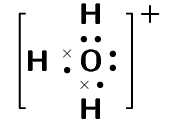sulfur dioxide ($$\text{SO}_{2}$$)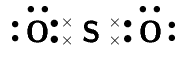Two chemical reactions are described below.

• nitrogen and hydrogen react to form $$\text{NH}_{3}$$
• carbon and hydrogen bond to form $$\text{CH}_{4}$$

For each reaction, give:

the number of valence electrons for each of the atoms involved in the reaction

Nitrogen: $$\text{5}$$, hydrogen: $$\text{1}$$ Carbon: $$\text{4}$$, hydrogen: $$\text{1}$$

the Lewis diagram of the product that is formed

$$\text{NH}_{3}$$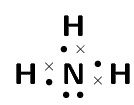$$\text{CH}_{4}$$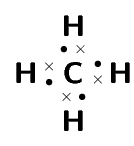the name of the product

$$\text{NH}_{3}$$: ammonia

$$\text{CH}_{4}$$: methane

A chemical compound has the following Lewis diagram:How many valence electrons does element Y have?

$$\text{6}$$. There are $$\text{6}$$ dots around element Y and from our knowledge of Lewis diagrams we know that these represent the valence electrons.

How many valence electrons does element X have?

$$\text{1}$$. X contributes one electron (represented by a cross) to the bond and X has no other electrons.

How many covalent bonds are in the molecule?

$$\text{2}$$ single bonds. From our knowledge of Lewis diagrams we look at how many cross and dot pairs there are in the molecule and that gives us the number of covalent bonds.

These are single bonds since there is only one dot and cross pair between adjacent atoms.

Suggest a name for the elements X and Y.

The most likely atoms are: Y: oxygen and X: hydrogen.

Note that Y could also be sulfur and X hydrogen and the molecule would then be hydrogen sulfide (sulfur dihydride).

Complete the following table:

 Compound $$\text{CO}_{2}$$ $$\text{CF}_{4}$$ $$\text{HI}$$ $$\text{C}_{2}\text{H}_{2}$$ Lewis diagram Total number of bonding pairs Total number of non-bonding pairs Single, double or triple bonds
 Compound $$\text{CO}_{2}$$ $$\text{CF}_{4}$$ $$\text{HI}$$ $$\text{C}_{2}\text{H}_{2}$$ Lewis diagram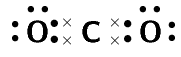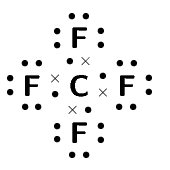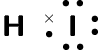Total number of bonding pairs $$\text{4}$$ $$\text{4}$$ $$\text{1}$$ $$\text{5}$$ Total number of non-bonding pairs $$\text{4}$$ $$\text{12}$$ $$\text{3}$$ $$\text{0}$$ Single, double or triple bonds Two double bonds Four single bonds One single bond One triple bond and two single bonds